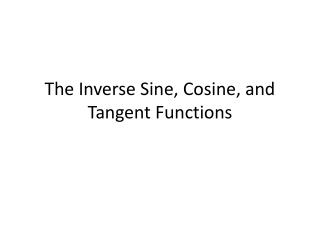DownloadDownload PresentationThe Inverse Sine, Cosine, and Tangent Functions

# The Inverse Sine, Cosine, and Tangent Functions

Télécharger la présentation## The Inverse Sine, Cosine, and Tangent Functions

- - - - - - - - - - - - - - - - - - - - - - - - - - - E N D - - - - - - - - - - - - - - - - - - - - - - - - - - -
##### Presentation Transcript

1. The Inverse Sine, Cosine, and Tangent Functions

2. 5. Use a calculator to find the value of each expression rounded to two decimal places.(Similar to p.192 #26)

3. 6. Use a calculator to find the value of each expression rounded to two decimal places.(Similar to p.192 #32)

4. 7. Find the exact value of each expression. Do not use a calculator(Similar to p.192 #38)

5. 8. Find the exact value of each expression. Do not use a calculator.(Similar to p.192 #44)

6. 9. Find the exact value, if any, of each composite function. If there is no value, say it is “not defined”. Do not use a calculator.(Similar to p.192 #46)

7. 10. Find the exact value, if any, of each composite function. If there is no value, say it is “not defined”. Do not use a calculator.(Similar to p.192 #50)

8. 11. Find the inverse function f-1 of each function f.(Similar to p.192 #54)